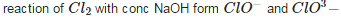Courses

# Some P-Block Elements - Practice Test (1)

## 25 Questions MCQ Test Additional Question Bank | Some P-Block Elements - Practice Test (1)

Description
This mock test of Some P-Block Elements - Practice Test (1) for Class 9 helps you for every Class 9 entrance exam. This contains 25 Multiple Choice Questions for Class 9 Some P-Block Elements - Practice Test (1) (mcq) to study with solutions a complete question bank. The solved questions answers in this Some P-Block Elements - Practice Test (1) quiz give you a good mix of easy questions and tough questions. Class 9 students definitely take this Some P-Block Elements - Practice Test (1) exercise for a better result in the exam. You can find other Some P-Block Elements - Practice Test (1) extra questions, long questions & short questions for Class 9 on EduRev as well by searching above.
QUESTION: 1

### The valence shell electronic configuration of p-block elements is ns2np1-6. But this is not true in the case of

Solution:

He has 1s2 configuration. It is a noble gas.

QUESTION: 2

### The angular shape of ozone molecules (O3) consists of

Solution:

Oconsists of 2 sigma bond and 1 pie bond.

QUESTION: 3

### Catenation i.e., linking of similar atoms depends on size and electronic configuration of atoms. The tendency of catenation in Group 14 elements follows the order:

Solution:

Catenation property decreases down the group

QUESTION: 4

Regular use of which of the following fertilizers increases the acidity of the soil?

Solution:

Ammonium sulphate addition increases acidity in the soil.

QUESTION: 5

Among the following which is the strongest oxidizing agent?

Solution:

F2 is very strong oxidizing agent.

QUESTION: 6

There are six groups of p-block elements in the periodic table numbering from

Solution:

P block elements starts from group 13 to group 18.

QUESTION: 7

Argon is used

Solution:

Ar is used in high temp welding.

QUESTION: 8

In the structure of diborane

Solution:

B2H6 has 4 terminal H attached to B atoms ans all these lie I the same plane. Also in diborane we have 2 bridging hydrogen(3c 2 electron ) which lie perpendicular to the plane.

QUESTION: 9

The percentage of p-character in the orbitals forming P - P bonds in P4 is

Solution:

obitals forming P-P bond is sp3 hybridised therefore have 75% p character.

QUESTION: 10

The brown ring test for nitrates depends on

Solution:

in ring test of nitrates nitrate ion is reduced to nitric oxide.

QUESTION: 11

The variation in properties in a group of p-block of elements is due to

Solution:

The difference in properties of p block element is because of presence of inner lying d and f electrons.

QUESTION: 12

Silicon is the second most abundant element on the earth’s crust and is a component of

Solution:

Si is the most imp component of ceramics.

QUESTION: 13

Quartz is extensively used as a piezoelectric material, it contains ___________.

Solution:

Quartz contains Si.

QUESTION: 14

When Cl2 gas reacts with hot and concentrated sodium hydroxide solution, the oxidation number of chlorine changes from

Solution:QUESTION: 15

One mole of magnesium nitride on the reaction with an excess of water gives:

Solution:

Mg3N2 on hydrolysis roduces two moles of ammonia.

QUESTION: 16

The maximum oxidation state shown by a p-block element is

Solution:

Maximum oxidation state of any p block element is sum of s and p electrons present in valence shell. Example for group 15 elements valence shell configuration is ns2np3 so max oxidation state is +5

QUESTION: 17

Carbon is most widely distributed in nature. In combined state it is available as

Solution:

In combined C can occur like hydrocarbons.

QUESTION: 18

The linear shape of CO2 is due to _________.

Solution:

O=C=O in CO2 hybridisation of C is sp so due to pЛ –pЛ bonding it is linear.

QUESTION: 19

In which of the following compounds, nitrogen exhibits highest oxidation state?

Solution:

In azide ( N3) have oxidation state -1/3

QUESTION: 20

Which of the following statements is not valid for oxoacids of phosphorus?

Solution:

hypophosphous acid is H3PO2 which is monoprotic acid.

QUESTION: 21

The sum of the s and p-electrons is called

Solution:

Max oxidation state is also known as group oxidation state.

QUESTION: 22

Stable isotopes of carbon are

Solution:

C12and C13 isotope of Carbon are very stable.

QUESTION: 23

The reason for small radius of Ga compared to Al is _______.

Solution:

due to screening effect size of Ga< Al as in Ga d-electrons are present which have poor shielding effect so nuclear charge increases and size decreases.

QUESTION: 24

The correct order of increasing bond angles in the following species is

Solution:

The correct order of increasing bond angles in the following species is Clo2< Cl2O < ClO2

QUESTION: 25

Argon is used

Solution:

Ar is used in high temp welding.